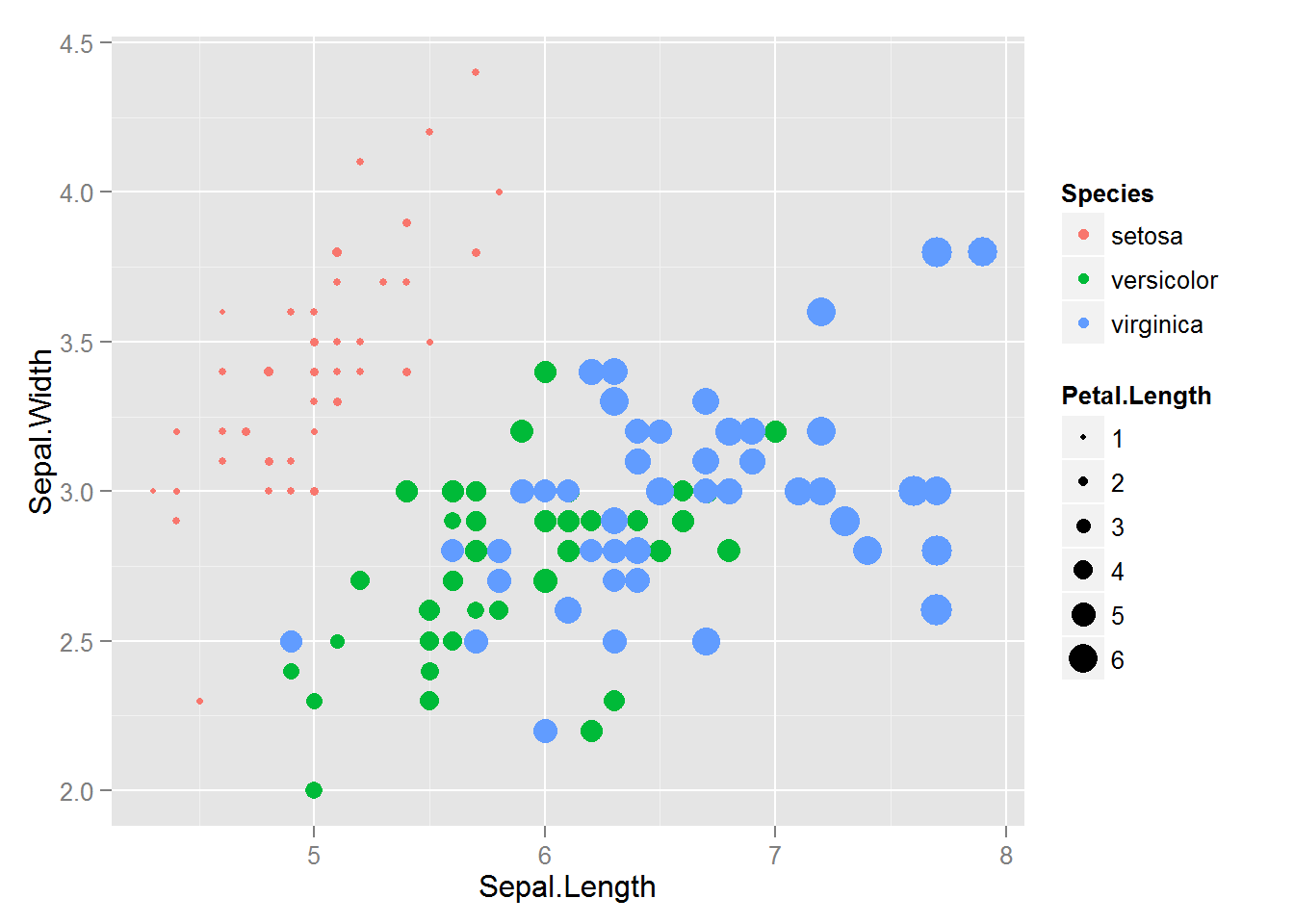# p value demonstration

We’ve got two short pieces of code to work with today.

https://www.dropbox.com/s/loawliymkl2tex7/p%20value%20demonstration.R?dl=1

## The first is the demonstration

#########################################
# PLAY WITH THE VALUES YOURSELF
#########################################
# set the probabilities for your set-up
alpha <- .05 # the probability of a sig result given that the null is true (confidence level)
beta <- .8 # the probability of a sig result given that the null is false (power)
p_null <- .5 # how likely is the null hypothesis to be true?

p_sig <- alpha*p_null + (1-beta)*(1-p_null) # the probability of a significant result (regardless of whether the null is true or not)

p_null_given_sig <- (alpha*p_null)/p_sig # the probability of the null hypothesis being true, given that you've got a sig result

## And the second is the plot

# see how your inferences about the null hypothesis following a sig result should change based on how probable the null was

betas_to_try <- c(.1, .3, .5, .7, .9) # the shape of that curve depends on the power of your experiment

plot.data <- data.frame(alpha=.05,
power_level=gl(k=15, n=length(betas_to_try), labels=c(betas_to_try)),
p_null=seq(from=0, to=1, length.out=15))

plot.data$beta <- as.numeric(as.character(plot.data$power_level)) # making a numeric version of the power factor, to use in the calculations below
plot.data$p_sig <- with(plot.data, alpha*p_null + (1-beta)*(1-p_null)) plot.data$p_null_given_sig <- with(plot.data, (alpha*p_null)/p_sig)

library(ggplot2)
ggplot(plot.data, aes(x=p_null, y=(1-p_null_given_sig), color=power_level)) +
geom_line() +
xlab("Probability of the null") +
ylab(NULL) +
ggtitle("What's the probabilty the null is false,\ngiven a significant result?")

# Interactive Embedded Plots with Plotly and ggplot2

Largley lifted from this r-bloggers post

install.packages("devtools")  # so we can install from GitHub
devtools::install_github("ropensci/plotly")  # plotly is part of rOpenSci
library(plotly)

py <- plotly(username="jflournoy", key="mg34ox914h")  # open plotly connection
# I'll change my key after this, but you can still use: plotly(username="r_user_guide", key="mw5isa4yqp")

gg <- ggplot(iris) +
geom_point(aes(Sepal.Length, Sepal.Width,color=Species,size=Petal.Length))
gg#This looks a little object-oriented like python
pyggplotly(gg) You can embed code like this (which you get from the plotly ‘share’ dialogue): <div> <a href="https://plot.ly/~jflournoy/16/" target="_blank" title="Sepal.Width vs Sepal.Length" style="display: block; text-align: center;"><img src="https://plot.ly/~jflournoy/16.png" alt="Sepal.Width vs Sepal.Length" style="max-width: 100%;width: 797px;" width="797" onerror="this.onerror=null;this.src='https://plot.ly/404.png';" /></a> <script data-plotly="jflournoy:16" src="https://plot.ly/embed.js" async></script> </div> You can also directly embed a plotly plot using a code chunk if you set plotly=TRUE for the chunk, and include session="knitr" in the call. #Set plotly=TRUE pyggplotly(gg, session="knitr")

There’s a wide world of plotly fun just waiting out there.

# Picking Pretty Plot Palates

The default colors for ggplot2 are pretty lovely, in my opinion, but sometimes you want more control. Or maybe you’re just procrastinating some less appealing work. Whatever the reason, sometimes you find yourself wanting to test new color palates for your plots. Here’s an easy way to do that. I’m using color brewer, which is an awesome color tool for all of your programming needs, not just R. It includes some palates that are specifically designed to be colorblind safe, printer friendly, and/or photocopier friendly.

library(ggplot2)
library(RColorBrewer)

# pick a palette
display.brewer.all()